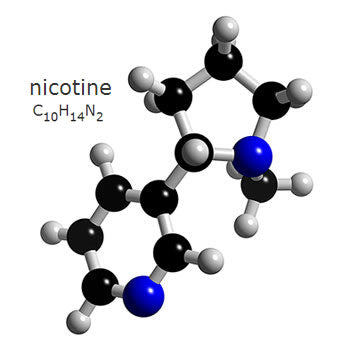## How much nicotine am I getting?It is confusing for new vapors to understand the terminology used for nicotine content.

Let’s start by talking about how we measure nicotine content. Most ejuice is measured in metric volumes. We use liters, milliliters (1/1000 of a liter), and milligrams (1/1000 of a milliliter). For us American vapers, this can make things a bit confusing from the start. Just keep in mind that one ounce is about 30 ml.

We measure nicotine as a ratio. In other words, we are looking for how much of a given volume consists of nicotine, versus other ingredients. There are two way the ratio is generally represented. Sometimes, you will see the ratio displayed as milligrams (mg) of nicotine per milliliter (ml) of fluid. As with Badger Juice, one of the following will be marked:

• 0 mg
• There are 0 milligrams of nicotine per milliliter of fluid.
• 6 mg
• There are 6 milligrams of nicotine per milliliter of fluid.
• 12 mg
• There are 12 milligrams of nicotine per milliliter of fluid.
• 18 mg
• There are 18 milligrams of nicotine per milliliter of fluid.
• 24 mg
• There are 24 milligrams of nicotine per milliliter of fluid.

So, if you want to know how much nicotine is in a bottle of juice, you can simply multiply the mg value by the bottle size in milliliters.

For example, 6 mg 10 ml bottle will contain a total of 60 mg of nicotine.

A 10 ml bottle of 24 mg would contain 240 mg of nicotine.

The other way you may see nicotine content displayed, is as a percentage of the total volume. Since there are 1000 mg in a milliliter, it is relatively simple to switch between ratios and percentages. 6 mg/ml would translate to 0.6% mg. In other words, 6/1000 of the total volume consists of nicotine. 24 mg would be 2.4% nicotine.

If you are looking for a simple way to estimate your daily nicotine consumption, we would simply look at how much ejuice you use in a day. For example, if you have a 2 ml tank, and you fill it each morning with 12 mg Badger Juice, you are consuming 24 mg of nicotine per day. If you have an Aspire Nautilus, and consume a full tank (5 ml) of 6 mg Badger Juice every day, you are consuming 30 mg.

For a better understanding of how this compares to analog cigarettes, see my recent post, How Much e-Juice Equals a Pack of Cigarette?

*this post originally appeared at the Vapor Cafe Blog